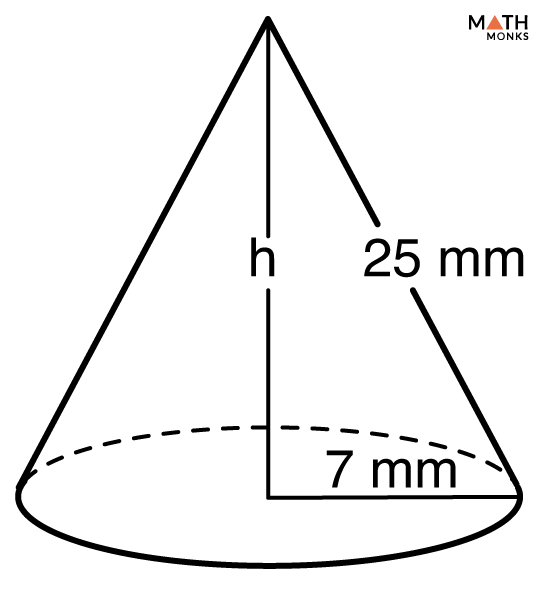# Volume of a Cone

The volume of a cone is the space it occupies in the three-dimensional plane. The volume is measured in cubic units such as m3, cm3, mm3, ft3, or in3.

Here we will discuss the volume of a right circular cone.

## Formulas

The basic formula to find the volume of a cone with height and radius is:

#### Derivation

We can relate the volume of a cone with that of a cylinder in the same way as the volume of a pyramid with that of a prism.

Let us consider a cylinder with a radius ‘r’ and height ‘h’. Now let us imagine a cone with the same base and height as the cylinder as in the diagram below.

∴ Radius of the cylinder = Radius of the cone = r

And, Height of the cylinder = Height of the cone = h

Also, Volume of a cylinder = πr2h

And, Volume of a cone = ${\left( V\right) =\dfrac{1}{3}\pi r^{2}h}$

Hence, the volume of a cone is one-third of that of a cylinder.

Find the volume of a right circular cone with a radius of 6 mm and height of 11 mm.

Solution:

As we know,
Volume ${\left( V\right) =\dfrac{1}{3}\pi r^{2}h}$, here r = 6 mm, h = 11 mm, π = 3.141
∴ ${V=\dfrac{1}{3}\times \pi \times 6^{2}\times 11}$
= 414.7 mm3

Find the Volume of an ice-cream cone having a radius of 18 mm and a height of 80 mm.

Solution:

As we know,
Volume ${\left( V\right) =\dfrac{1}{3}\pi r^{2}h}$, here r = 18 mm, h = 80 mm, π = 3.141
∴ ${V=\dfrac{1}{3}\times \pi \times 18^{2}\times 80}$
≈ 27143.36 mm3

Finding the volume of a cone with DIAMETER and HEIGHT

Calculate the volume of a cone with a diameter of 8 mm and height of 13 mm.

Solution:

As we know,
Volume ${\left( V\right) =\dfrac{1}{12}\pi d^{2}h}$, here d = 8 mm, h = 11mm, π = 3.141
∴ ${V=\dfrac{1}{12}\times \pi \times 8^{2}\times 13}$
= 217.82 mm3

Finding the VOLUME of a cone when the SLANT HEIGHT and RADIUS are known

Calculate the Volume of a cone with a slant height of 25 mm and a radius of 7 mm.

Solution:We have drawn the figure alongside as per the data given.
Here, we will apply the Pythagorean Theorem to find out the height ‘h’ of the cone.
h2 = s2 – r2, here s = 25 mm, r = 7 mm
∴ h2 = 252 – 72
h2 = 576
h = √576
= 24 mm
Now we will apply the direct formula:
Volume ${\left( V\right) =\dfrac{1}{3}\pi r^{2}h}$, here r = 7 mm, h = 24mm, π = 3.141
∴ ${V=\dfrac{1}{3}\times \pi \times 7^{2}\times 24}$
= 1231.5 mm3

A cone can be truncated. Such a cone is also known as a frustum of a cone. We can calculate the volume of such cones in the chapter, volume of a frustum of a cone.